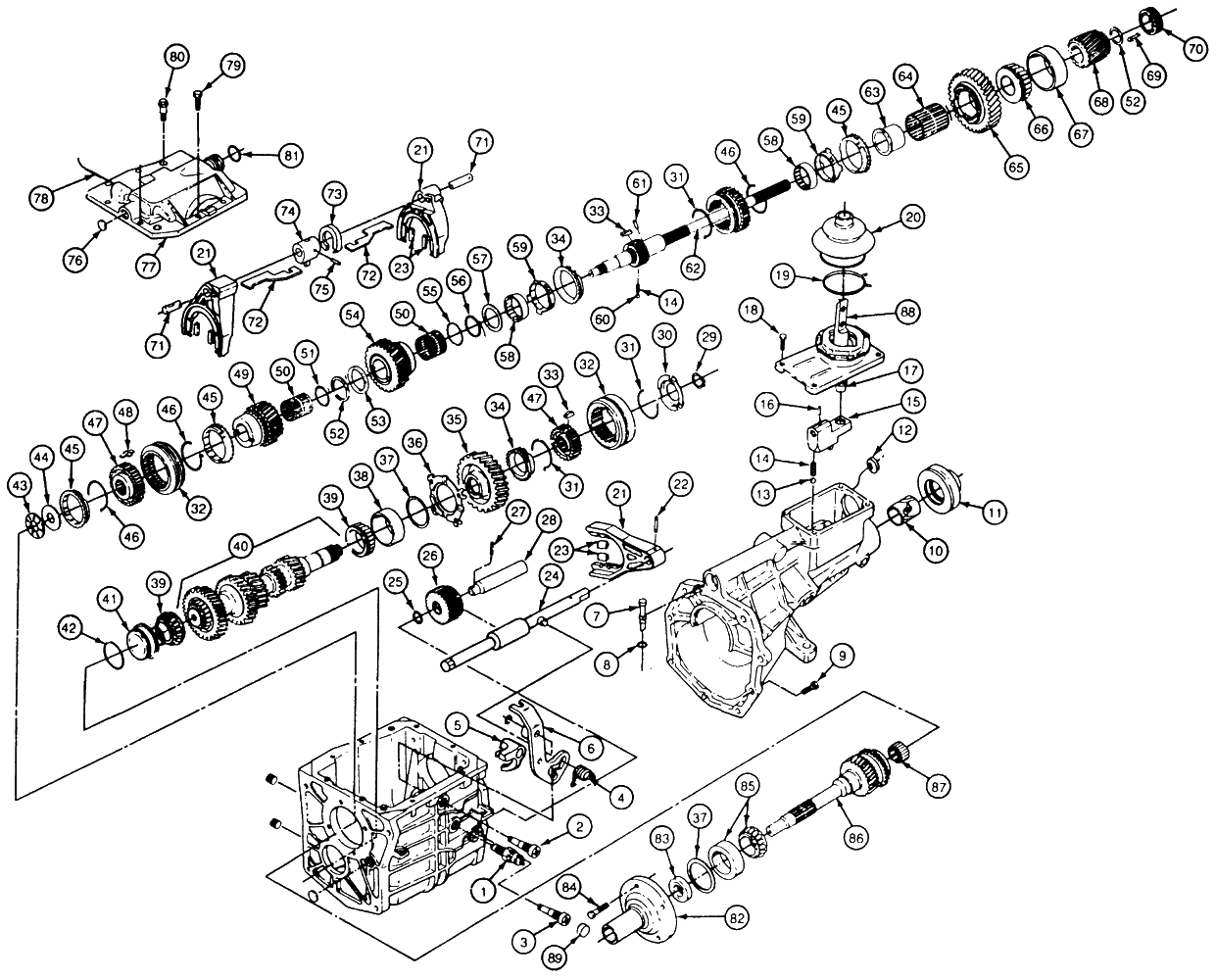# Antescofo Library of Predefined FunctionsAntescofo includes a set of predefined functions. They are described mostly in the chapters Expressions, Scalar values and Data structures. For the reader's convenience, we give here a list of these functions.

In the following pages, the sequence of names after the function defines the type of the arguments accepted by a function. For example numeric is used when an argument must satisfy the predicate @is_numeric, that is @is_int or @is_float. In addition, we use the term value when the function accepts any kind of argument.

### Listable Functions and Listable Predicates¶

When a function f is marked as listable, the function is extended to accept tab arguments in addition to scalar arguments. Usually, the result of the application of f on a tab is the tab resulting on the point-wise application of f to the scalar elements of the tab. But for predicate, i.e. a function that returns a bool, the result is the predicate that returns true if the scalar version returns true on all the elements of the tabs.

For example, @abs is a listable function on numeric, so it can be applied to a tab of numerics. The result is the tab of the absolute value of the elements of the tab argument.

Another example: the function @approx is a listable predicate and @approx(u, v) returns true if @approx(u[i], v[i]) returns true for all elements i of the tabs u and v.

### Overloaded functions¶

Some functions accept different kinds of values for the same argument. For example @insert accepts tab or map as its first argument. Such functions are said overloaded: they gather under the same name several specialized version of the function.

A user-defined function can be overloaded: it recquires to check the type of the argument value to dispatch to the specialized versions (written elsewhere).

Overloading methods is more simple: the same identifier can be used to name a method in different objects.

### Side-Effect¶

Most functions are pure functions, that is, they do not modify their arguments and build a new value for the result.

In some cases, the function works by a side-effect, like @push_back which modifies its argument in place. Such functions are marked as impure. We also qualify functions that may return different values when called with the same arguments as impure, even if they do not produce a side-effect (for example, functions that return a random number).

### Special forms¶

Special forms are syntactic constructs similar to function calls but that are subject to some restriction or that behave differently of a function call.

For example, boolean predicates are a special form of function application because they do not always evaluate all their arguments (they are lazy). For instance, the conjunction && evaluates its second argument only if the first is not false.

Another example is @plot, @history_length or @dumpvar that only accepts a variable as an argument, not a general expression.

Special forms cannot be curryfied nor passed as an argument: they are not ordinary functional values. Note, howevever that they can be wrapped in a user-defined function which is an ordinary functional value.

### Infix function call¶

A function call is usually written in prefix form:

      @drop($t, 1) @scramble($t)


It is possible to write function calls in infix form, as follows:

      $t.@drop(1)$t.@scramble()


The @ character is optional when naming a function in infix call, so we can also write:

      $t.drop(1)$t.scramble()


This syntax is the same as for a method call. The general form is:

      arg1 . @fct (arg2, arg3, ...)    ; or more simply
arg1 . fct (arg2, arg3, ...)


The argi are expressions. Notice that the infix call, with or without the @ in the function name, is not ambiguous with the notation used to refer to a variable local $x in a compound action from the exe of this action, exe.$x, because $x cannot be the name of a function. The infix notation is less general than the prefix notation. In the prefix notation, the function can be given by an expression. For example, functions can be stored into an array and then called following the result of an expression: $t := [@f, @g]
; ...
(\$t[exp])()


will call @f or @g following the value returned by the evaluation of exp. Only function name (with or without @) are accepted in the infix notation.

## Listing by categories¶

### Mathematical Functions¶

in addition, see listable functions.

### Listable Functions¶

@!=,    @%,    @&&,    @!=,    @%,    @&&,    @*,    @+,    @-,    @<,    @<=,    @==,    @>,    @>=,    @div,    @||,    @+,    @-,    @<,    @<=,    @==,    @>,    @>=,    @div,    @||,    @/,    @abs,    @acos,    @approx,    @asin,    @atan,    @atan2    @between,    @ceil,    @cos,    @cosh,    @exp,    @floor,    @log,    @log10,    @log2,    @pow,    @round,    @sin,    @sinh,    @sqrt,    @tan